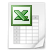### Panmagic 4x4 inlay square inside a 6x6 magic square

An inlay square is a (not pure) magic square inside a larger (pure) magic square.

The smallest even inlay square is a (not pure) 4x4 square inside a (pure) 6x6 square. In a pure 6x6 square are the numbers from 1 up to 36.

 1 2 3 4 5 6 7 8 9 10 11 12 13 14 15 16 17 18 19 20 21 22 23 24 25 26

 27 28 29 30 31 32 33 34 35 36 -10 -9 -8 -7 -6 -5 -4 -3 -2 -1

To construct the 4x4 inlay square, use the middle 16 numbers (= yellow marked). Take for example the first basic panmagic 4x4 square and add 10 to each number.

Pure 4x4 +10           =   (not pure) inlay 4x4

 1 8 13 12 11 18 23 22 15 10 3 6 25 20 13 16 4 5 16 9 14 15 26 19 14 11 2 7 24 21 12 17

There are the following three boundary conditions to construct the border of the pure 6x6 magic square:

A positive number in each row/column/diagonal must be put opposite the same negative number (= translation of the numbers 27 up to 36);

In the top row, the bottom row, the left column and the right column must be put 3x positive and 3x negative number;

● The numbers of the top row, the bottom row, the left column and the right column must each time sum to 0.

Make the top row and the right column and start top left (for example) with the number 1. Because the number top left is 1, the number -1 must be put right down. The number to be filled in top right (?) is as well in the top row as the right column.

 1 ? -1

The sum of 1 up to 10 is 55. You need the numbers 1 up to 10 plus two numbers double. Take the numbers 1 and 8 double, than the sum of each 3 numbers must be: [55 + 1 + 8] / 4 = 16.

1+5+10

1+6+ 9

1+7+ 8

2+4+10

2+5+ 9

2+6+ 8

3+4+ 9

3+5+ 8

3+6+ 7

4+5+ 7

Above I puzzled 4x 3 possibilities. The numbers can be filled in as follows.

Fill in 4 possibilities                      Fill in opposite numbers

 1 6 9 -3 -5 -8 1 6 9 -3 -5 -8 2 -2 2 4 -4 4 10 -10 10 -7 7 -7 -1 8 -6 -9 3 5 -1

The result is:

 1 6 9 34 32 29 35 11 18 23 22 2 33 25 20 13 16 4 27 14 15 26 19 10 7 24 21 12 17 30 8 31 28 3 5 36

Use this method to construct inlaid magic squares of even order. See6x6, Panmagic 4x4 in 6x6.xls